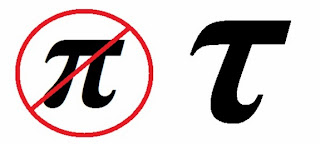Friday, July 8, 2011

Get rid of pi?

If math bores you to death, this post is not for you, and I apologize in advance.  However, I feel that I'm allowed a math post every now and then.  Furthermore, Brett's the one who showed me all this, so you can blame him.

So, here's the thing.  You know pi, that wonderful irrational number that's approximately 3.14?  Well, some mathematicians are suggesting we get rid of it.  I know, I know.  Mathematical blasphemy, right?

Maybe not...The suggestion is that we don't just get rid of pi, but we replace it with tau, which will equal 2pi.

So...this is what brilliant minds have come up with?  Let's just take a number we've been using for centuries and multiply it by two?

At first it sounds crazy, I admit.  But, the more I think about it, the more it makes sense.  The most basic (and, in my opinion, most beautiful) argument for switching from pi to tau is the unit circle.  What's the unit circle, you ask?  Basically, it sums up everything you learn in trig, except maybe identities.  As one of my professors says, "There's nothing left to be discovered in trigonometry.  Draw that circle, and you're done."  Typically, we use radians instead of degrees to measure the angles inside this circle.  Instead of saying a full rotation on the circle is 360 degrees, we say it's equal to 2pi.  Weird, I know.  So, the unit circle is divided up like this:

Do you notice the oddity?  A quarter of the circle isn't a quarter of pi, it's a half of pi...a half of the circle isn't half of pi, it's just pi.  You can see how this can be confusing to students.

However, if we switched to tau, the unit circle would look like this:

Makes much more sense, right?  Now a quarter of the circle is a quarter of tau, etc, etc.

If I ever get to teach trig, I will certainly introduce this new constant which is equal to the ratio of a circle's circumference over its radius, as opposed to the circle's circumference over its diameter. (Where did we get that??  When do we ever use the diameter?)

If you're interested in learning more, I highly recommend this article.  If you consider yourself pretty mathy, you should definitely read the entire Tau Manifesto, which gives many more arguments for the conversion than the one I just described.  If you're into connections between math and music, check out What Tau Sounds Like.

For those still reading, thanks for hanging in there.

Long live Tau.

1 comment:

1.Hmm... I must admit that even though I'm not the math fan that you are, I rather like the idea of tau.